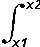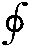# Mathematical Symbols: math notation list / chart

## Many math / mathematical operators, symbols & notations are used in scientific & maths equations.

Letter & Symbols Includes:
Greek alphabet     Mathematical symbols     Mathematical constants     Typographical symbols

To concisely signify different mathematical operations a variety of signs and symbols are used. These mathematical operators and symbols range from simple addition and subtraction to more complicated operations like integration and the like.

There are many math notations and symbols that are in common use - the list below gives some of the more widely used symbols and notations.

Mathematical Symbols List

Mathematical
Symbols
Name
Meaning
Explanation / example
of Mathematical Symbols
+ Plus
The addition of two or more quantities, e.g. 2 + 3 = 5
- Minus This represents the subtraction of one quantity from another, e.g. 3 - 2 = 1
× Multiplication, multiply This operator represents the multiplication of two quantities, e.g. 3 × 2 = 6
* Multiplication The * is the same as the x multiplication sign, but it is often used in computer terminology as a result of possible confusion with the letter 'x'.
Multiplication The ⋅ is the same as the × multiplication sign, but it is often used in mathematical notations to prevent possible confusion with the letter 'x'. e.g. y × x is often written as y ⋅ x.
÷ Division, divide This is used to indicate that one number is divided by another, e.g. 3 ÷ 2 = 1.5
/ Division, divide This is used instead of the ÷ character which is not widely used in computer characters. An example would be 3 / 2 = 5, and it represents the format of fractions.
= Equals x = y means that x and y are equal and represent the same value.
Not equal to x ≠ y means that x and y are not the same and do not represent the same value, e.g. 3 ≠ 4.
Therefore, hence Sometimes used in proofs before logical consequences
~ Similar to m ~ n means the quantities m and n have the same order of magnitude, or general size, e.g. 110 ~ 111.
Almost equal x ≈ y means x is approximately equal to y, e.g. 110 ≈ 110.112233
Congruent to
Sum This sign is used where a sum of quantities is required, The range over which the sum takes places are normally marked at the bottom and top right hand sides of the ∑ sign.
Equivalent This sign is used to represent equivalence. The two items that are equivalent would not be directly equal.
Square root This is used to indicate the square root of a number, e.g. √2 = 1.414Integral This is used to indicate that an equation is integrated. The range over which the integration takes places are normally marked at the bottom and top right hand sides of the ∫ sign. For example ∫ f(x) dx represents a function whose derivative is f.Contour integral Similar to the standard integral, but this mathematical symbol is used to denote a single integration over a contour, i.e. a closed curve or loop.
δ delta
Proportional This symbol is used to indicate proportionality, possibly to show that when one figure doubles so does the other. For example y ∝ x when y = k ⋅ x
Infinity
AND Used to indicate the Boolean "AND" operator
OR Used to indicate the Boolean "OR" operator
Angle Used to indicate an angle. It may be used in a variety of ways, possibly along with a figure to show it represents an angle.
Perpendicular Used to indicate that two lines are perpendicular to each other.
± Plus or minus. Used in a variety of ways to indicate that the figure could be plus or minus. It is often used to provide a range, e.g. 10 ± 2 or equivalently 10 ± 20% means the range from 10 ? 2 to 10 + 2, or 10 + 20% or 10 - 20%.

The use of mathematical or math notations and symbols along with some straightforward rules enables mathematical operations to be expressed in a logical form and provides for an environment where there is no room for ambiguity of what is intended.

As a result, these math operators and notations are used universally around the globe.

More Basic Electronics Concepts & Tutorials:
Voltage     Current     Power     Resistance     Capacitance     Inductance     Transformers     Decibel, dB     Kirchoff's Laws     Q, quality factor     RF noise     Waveforms